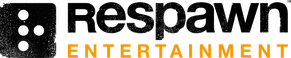Respawn Entertainment, LLC was founded April, 2010 by Jason West and Vince Zampella. A big portion of Respawn is made up of ex-Infinity Ward staffers, who followed the founding duo after the two were fired by Activision.

# Employees

Person MoH:AA COD COD2 COD4 MW2 TF TF2
Abbie Heppe x
Alex Roycewicz x x x x
Alicia Alunni x
Amy Fry
Arturo Castro
Austin Arnett x x
Brad Allen x x x x x x x
Brandon Kelch
Brandon Mancilla x
Brent McLeod x x x x x
Brian Burbell x
Bruce Ferriz x x x
Bruno Werkneck
Carlos Pineda x
Carlos Rivera x
Chad Barb x x x x x
Chad Grenier x x x x x x
Cheng Lor x x x x
Chin Xian Chong x x
Chris Dionne x x x
Chris Hughes x x
Chris Lambert x x
Chris Shelton x
Chris Sutton
Chuck Wilson x x
Cliff Childs x
Danny Gardner x
David Brumbley x
David Nazario x
David Shaver x x
David Wightman
David Standley x
Dom McCarthy x x
Doug Lopez
Drew McCoy x x x x
Dusty Welch x x x x x
Earl Hammon x x x x x x x
Erik Kraber x x x
Ethan Ayer x
Fabrice Odero
Felipe Lerma x x
Frank Gigliotti x x x x x ?
Geoff Smith x x x x x
Griffin Dean x
Hanno Hagedorn
Hethe Srodawa x
Jaehyup Keum
Jake Keating x x x x
Jake Virginia x x
Jason Burton x
Jason De Heras
Jason Hill
Jason McCord x x x
Jason West x x x x x x
Jay Frechette x
Jean-Francois Rey
Jeff Magers
Jeremy Thurman x
Jiesang Song x x x x
Jimmie Harlow
Joe Lubertazzi x
Joel Conger x x
Joel Emslie x x x x x
Joel Gompert x x x x x
John Haggerty x x x x
John Lee
Jolenna Johnson
Jon Davis x x x x
Jon Shiring x x x x x
Jordan Lamarre-Wan
Jose Zavala x x
Josh Dunnam x x
Josh Medina x x
Joshua Nelson x x
Jung Park x
Justin Cook x
Justin Hendry x x
Ken Feldman
Kevin Anderson x x
Kevin Kim
Kristen Altamirano x
Kristin Christopher x x x x
Kyle Fujita x x
Laure Retif x
Leanne Garand x
Lewis Walden x x
Logan Miller x
Mackey McCandlish x x x x x x x
Mario Perez x x x
Mark Grigsby x x x x x
Mark Grimenstein x
Mike Altamirano x x
Mike Durnhofer x
Mike Kalas x x
Miriam Anderson x
Mitchell Norris
Mohammad Alavi x x x x x
Moy Parra x
Nathan Tullis x x
Nick Laviers x
Paul Barfield
Paul Messerly x x x x x x x
Preston Glenn x x x x x x x
Prog Wang
Ranon Sarono x
Rayme Vinson x x x x
Regie Santiago x
Richard Baker x x x x x
Richard Greenspan
Rick Grubel
Rick Hernandez x
Robb Shoberg x x
Robert Gaines x x x x x
Robert Bloss
Robert Taube x x
Robert Wilinski x
Rodrigo Ribeiro x
Roger Abrahamsson x x x x x
Roger Hughston x
Roni Papouban x x
Ryan Ehrenberg x x
Ryan Hakik x x
Ryan Lastimosa x x x x
Ryan Redetzke x x
Sean Bae
Sean Slayback x x x
Shane Holloway
Shawn Wilson x
Steve Fukuda x x x x x x x
Steve Johnson
Steve Marton
Steven Derose x x
Steven Mitchell x x x
Steven Wong x x
Stig Asmussen
Taylor West
Tim Shanks x x x
Todd Alderman x x x x x x x
Todd Sue x x x x
Tragan Monaghan x
Tri Do
Tu Bui x x
Tyler Parsons x x
Victor Durling x
Vince Zampella x x x x x x x
Will Cho x x x
Wonjae Kim x x
Xin Liu x
Zied Rieke x x x x x x